- Click to visit the Maths Genie
- Remember Mathsgrader usually only marks correct or partially correct answers but sometimes it misses a correct answer - any queries email ross@mathsgrader.com
- If you want to type a fraction like \frac{1}{2}, type 1/2.
- If you want to type x^2 type x^2.

Wk3 Assessment Tier C

Q1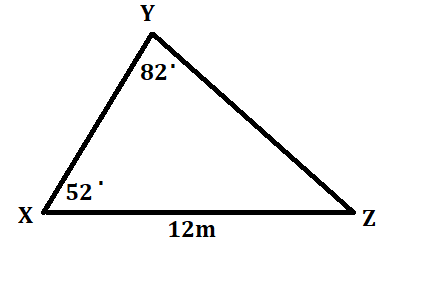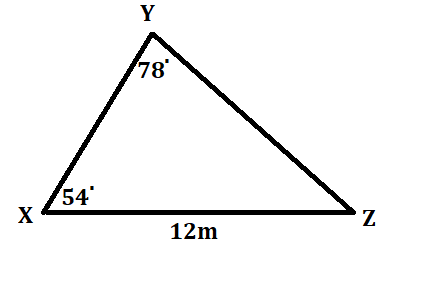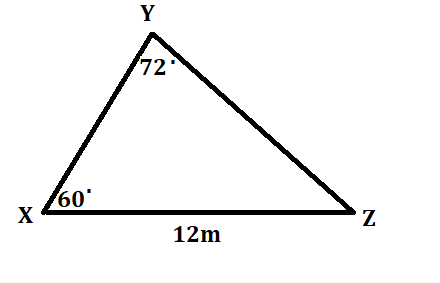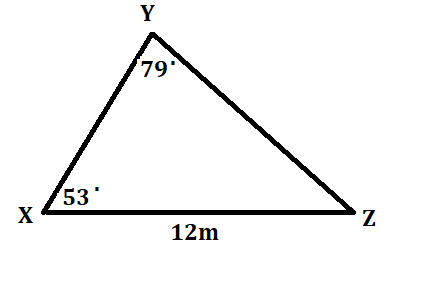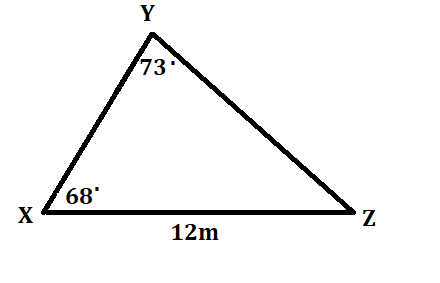a)
m
(2 marks)

b)
m^2
(3 marks)

Q2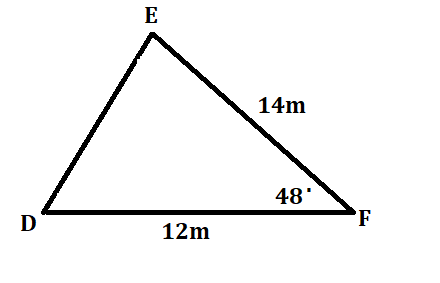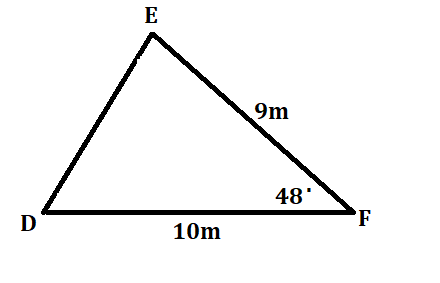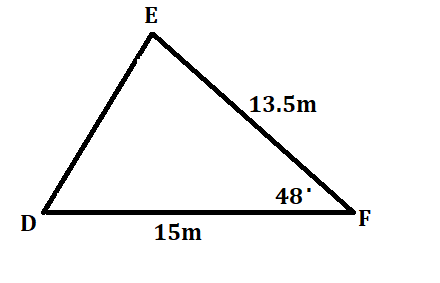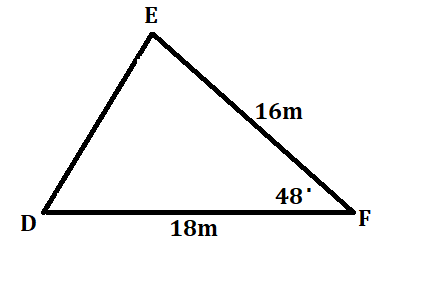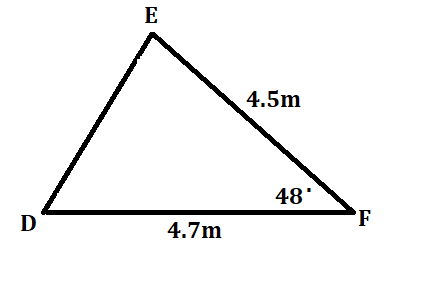i)

x= m
(2 marks)

ii)

x= m^2
(2 marks)

Q3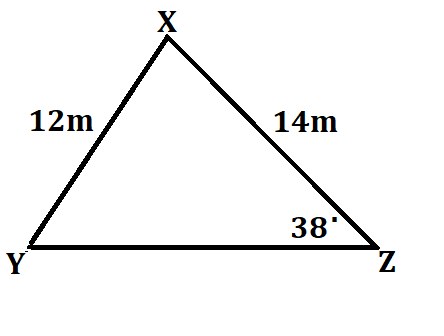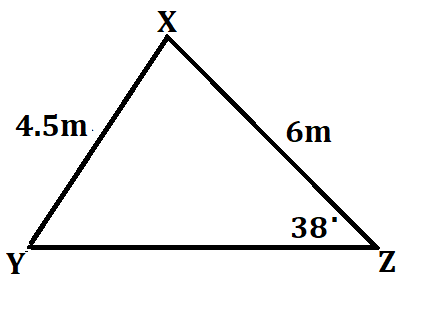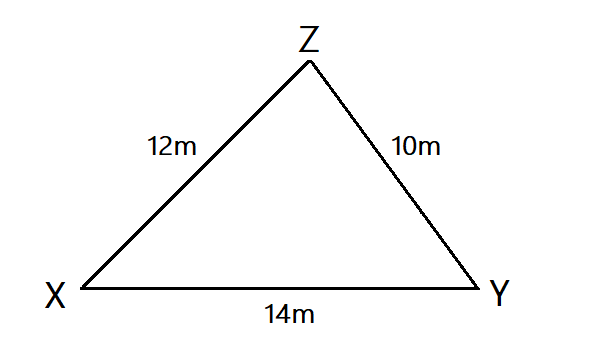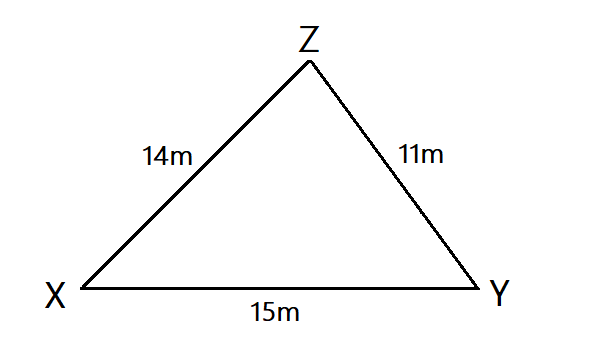degrees
(3 marks)

Q4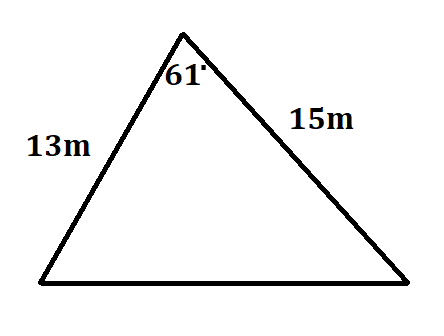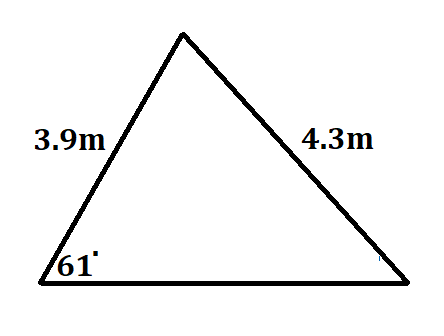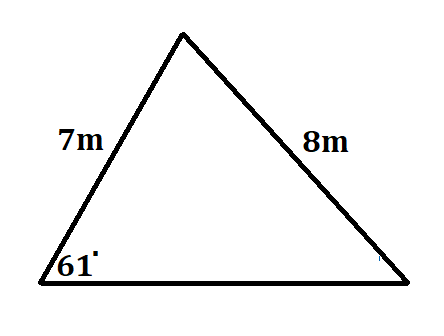m
(4 marks)

Q5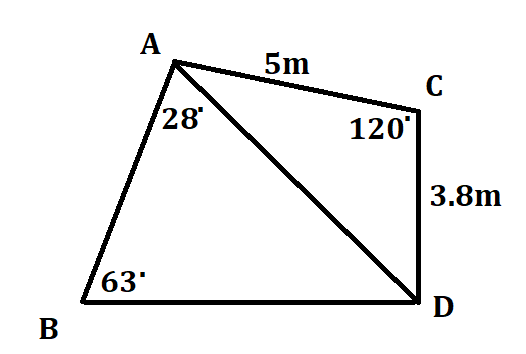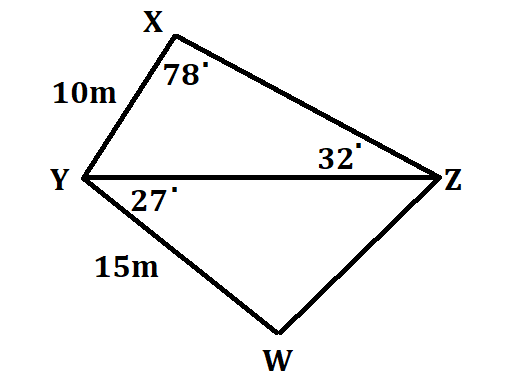m
(4 marks)